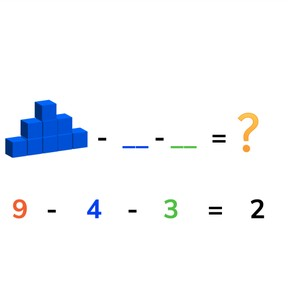Subtraction to 10 with three numbers

# Subtraction to 10 with three numbers8,000 schools use Gynzy92,000 teachers use Gynzy1,600,000 students use Gynzy

## General

Students learn to subtract three numbers to 10.

1.OA.A
1.OA.C.5
1.OA.C.6

## Relevance

IT is important to be able to subtract two numbers from a total number. In some situations it is required, like when you are handing out candies and want to know how many you are left with in total.

## Introduction

Practice with two subtraction problems with the students on the interactive whiteboard. The subtraction problems are given in story form with visual support.

## Development

Start by discussing the importance of being able to subtract with three numbers, giving the practical example of needing to hand out candies to more than one person and wanting to know how many you have left. Next, explain to students how to solve a subtraction problem with multiple numbers (subtrahends) using blocks. Tell the students to first look at how many there are in total. This is the first number (minuend) of the subtraction problem. Then take the next number (subtrahend) and subtract that from the first number. Use that difference and subtract the final number (subtrahend) from the intermediate difference. Discuss with students that the order of the second and third numbers (subtrahends) does not matter, as long as the first number(minuend) stays in the same place at the start of the subtraction problem. Different methods to solve these subtraction problems follow on the interactive whiteboard like using the rekenrek or blocks. Discuss these as a class.

Check that your students understand subtraction to 10 with three numbers by asking the following questions:
- Why is it useful to be able to subtract multiple numbers from an original starting number?
- How do you subtract a subtraction problem with three numbers?
- Why can it be useful to swap the order of the second and third numbers (subtrahends) in a subtraction problem?

## Guided practice

Students first practice with a subtraction problem that starts at 5, followed by a problem starting at 7 and then at 9.

## Closing

Discuss with students what they think of subtraction with three numbers. Practice with two subtraction problems with visual support and then close with a game. Divide the class into groups. One student must stand in the middle of the group and comes up with a subtraction problem with three numbers. They spin in the middle with their eyes closed, pointing at their group members. When they stop spinning, the student they are pointing at must find the difference. If the answer given is correct, that student moves to the middle.

## Teaching tips

Students who have difficulty with this can be supported by the use of manipulatives or MAB blocks.

## Instruction materials

Optional: MAB blocks or other manipulatives

### The online teaching platform for interactive whiteboards and displays in schools

• Save time building lessons

• Manage the classroom more efficiently

• Increase student engagement